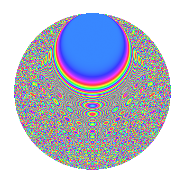# Properties

 Label 1050.2.bnLevel 1050 Weight 2 Character orbit bn Rep. character $$\chi_{1050}(131,\cdot)$$ Character field $$\Q(\zeta_{30})$$ Dimension 640 Sturm bound 480

# Related objects

## Defining parameters

 Level: $$N$$ = $$1050 = 2 \cdot 3 \cdot 5^{2} \cdot 7$$ Weight: $$k$$ = $$2$$ Character orbit: $$[\chi]$$ = 1050.bn (of order $$30$$ and degree $$8$$) Character conductor: $$\operatorname{cond}(\chi)$$ = $$525$$ Character field: $$\Q(\zeta_{30})$$ Sturm bound: $$480$$

## Dimensions

The following table gives the dimensions of various subspaces of $$M_{2}(1050, [\chi])$$.

Total New Old
Modular forms 1984 640 1344
Cusp forms 1856 640 1216
Eisenstein series 128 0 128

## Trace form

 $$640q - 80q^{4} + 4q^{7} + O(q^{10})$$ $$640q - 80q^{4} + 4q^{7} + 6q^{10} - 44q^{15} + 80q^{16} + 8q^{18} + 12q^{21} + 16q^{22} - 2q^{25} + 6q^{28} + 2q^{30} + 18q^{31} - 90q^{33} + 8q^{37} + 32q^{39} + 6q^{40} + 8q^{42} + 64q^{43} + 114q^{45} - 12q^{46} - 28q^{49} - 12q^{51} - 88q^{57} - 28q^{58} - 2q^{60} - 14q^{63} + 160q^{64} + 48q^{66} + 16q^{67} + 150q^{70} - 8q^{72} - 168q^{73} - 240q^{75} - 16q^{78} + 28q^{79} + 44q^{81} - 192q^{82} + 18q^{84} + 136q^{85} - 36q^{87} - 2q^{88} + 52q^{91} + 28q^{93} - 48q^{94} - 64q^{99} + O(q^{100})$$

## Decomposition of $$S_{2}^{\mathrm{new}}(1050, [\chi])$$ into newform subspaces

The newforms in this space have not yet been added to the LMFDB.

## Decomposition of $$S_{2}^{\mathrm{old}}(1050, [\chi])$$ into lower level spaces

$$S_{2}^{\mathrm{old}}(1050, [\chi]) \cong$$ $$S_{2}^{\mathrm{new}}(525, [\chi])$$$$^{\oplus 2}$$

## Hecke Characteristic Polynomials

There are no characteristic polynomials of Hecke operators in the database